Home > CC4 > Chapter A > Lesson A.1.8 > ProblemA-87

A-87.

Plot the points A(5, 3), B(−4, 3), C(−4, −6), and D(5, −6) on a set of axes. Connect the points in order. Then connect D to A to form a quadrilateral (a polygon with four sides). A-87 HW eTool (Desmos) Homework Help ✎

1. What kind of quadrilateral was formed?

2. How long is each side of the quadrilateral?

3. What is the area of the quadrilateral?

4. What is the perimeter of the quadrilateral?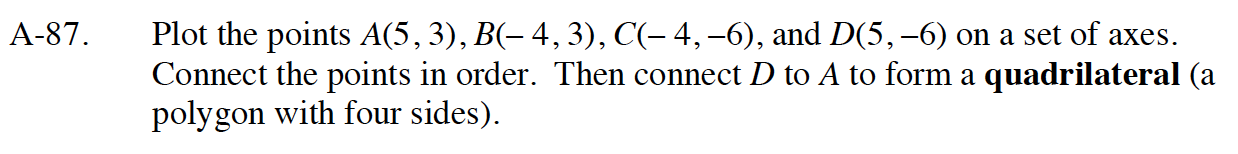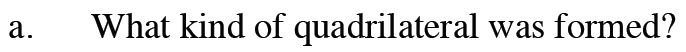Are there any congruent sides or angles?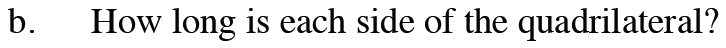Count the number of boxes from side to side.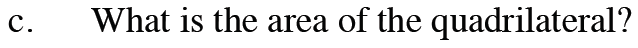Area = base x height.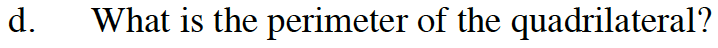Perimeter = sum of the side lengths.

Use the eTool below to solve each part.
Click the link at the right to view full eTool version: A-87 HW eTool.